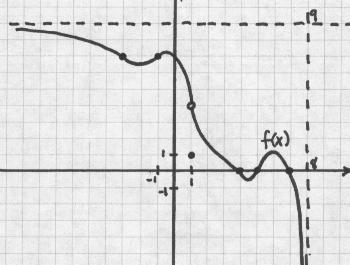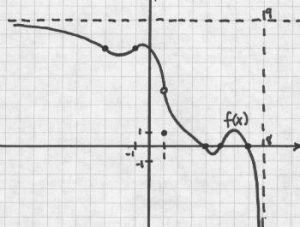# Problem 1: Limits

2011-2012 Describe or draw a function, f(x), with the following characteristics: f(x) has domain (–∞,8) f(x) has range (–∞,9) f(4) = 0; f(5) = 0; f(7) = 0 The limit, as x approaches –∞, of f(x) equals 9 The limit, as x approaches 8 from the left, of f(x) equals –∞ f(–1) = f(–3); f(–1) … Continue reading "Problem 1: Limits"## 2011-2012

Describe or draw a function, f(x), with the following characteristics:

• f(x) has domain (–∞,8)
• f(x) has range (–∞,9)
• f(4) = 0; f(5) = 0; f(7) = 0
• The limit, as x approaches –∞, of f(x) equals 9
• The limit, as x approaches 8 from the left, of f(x) equals –∞
• f(–1) = f(–3); f(–1) > f(–2)
• f(1) = 1
• The limit, as x approaches 1, of f(x) is 4

### One Solution (others possible but similar):The video may take a few seconds to load.Having trouble Viewing Video content? Some browsers do not support this version – Try a different browser.